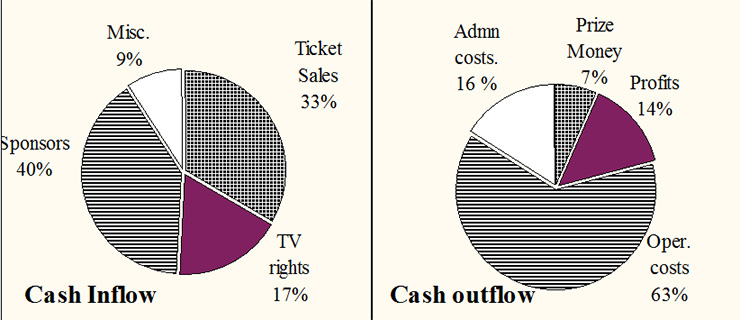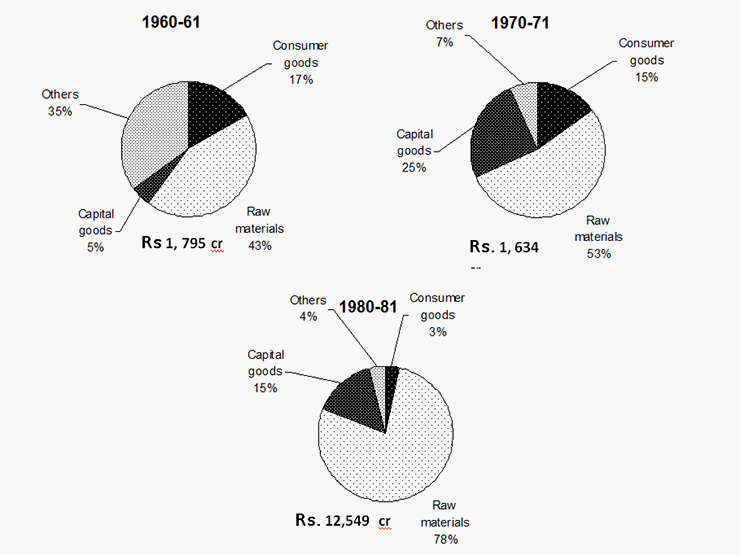# Pie Chart Practice Questions : Level 03

DIRECTIONS for questions 1 to 5: The following data is about the organization of the Nimbu Paani Cup. The event was jointly organized by the cricketing boards of India, Pakistan and Sri Lanka. The event was held between 01-Mar and 31-Mar.
Inflow = Outflow = Rs 120 cr
People Resources Involved:
 Players 180 Officials 1,400 Spectators 760,000 Security 17,000 Volunteers 14,0001. Profits among Sri Lanka, Pakistan and India Cricket boards are split in the ratio 1:2:3. The Board of Control for cricket in India (BCCI) pays a tax of 30%. Find the post tax profit of BCCI.
1. Rs 8.4 cr
2. Rs 2.8 cr
3. Rs 5.9 cr
4. Rs 16.8 cr
2. If 25% of operational costs can be attributed to security, then what is the average cost of a security personnel (Lakh)?
1. Rs 1.11
2. Rs 0.18
3. Rs 2.18
4. None of these
3. What was the average realization per spectator in the course of the games? (Assuming no overlaps of spectators)
1. Rs 435
2. Rs 521
3. Rs 400
4. None of these
4. Volunteers had been given tickets at subsidized rates of Rs 50. If they were charged the rates of 300, ticket sales would have changed by
1. Rs 42 lakhs
2. Rs 4.2 cr
3. Rs 35,000,000
4. None of these
5. Prize money as a %age of TV rights and sponsorship was approximately
1. 5.83
2. 8.4
3. 12
4. 14
DIRECTIONS for questions 6 to 10 : Refer to the following graph about Imports of various commodity groups.1. The import of Consumer Goods in 1960-61 cost how much Rs (in crore)?
1. 245
2. 108
3. 395
4. 305
2. What is the difference in the percentage of import of raw materials to the total from 1970-71 to 1980-81?
1. 10
2. 15
3. 17
4. None of these
3. By how many Rs (in crore) did the import of raw material increase from 1960-61 to 1970-71?
1. 65
2. 94
3. 150
4. 90
4. The value of import of capital goods during 1980-81 exceeded the value of the total imports in 1960-61 by how many crore?
1. Rs 108
2. Rs 100
3. Rs 87
4. Rs 90
5. From the 100000 tonnes of raw materials imported in 1970-71, nickel accounted for 200 tonnes. What was the price of Nickel in terms of Rs / ton?
1. 850
2. 8500
3. 85000
4. Cannot be determined
 Que. No. Answer Key Explanation 1. 3 Total profit = 14% of Rs 120 cr = 16.8 cr.; India’s share in profit = 3/6 × 16.8 cr = 8.4 cr Tax deduction = 30% of 8.4 cr = 2.52 cr; Post tax profit = 8.4 - 2.52 = Rs 5.9 cr 2. 4 Operational cost = 63% of 120 cr = 75.6 cr; 25% to security = 75.6 × (25/100) = 18.9 cr Avg. cost of a security personnel = 1890 lakh / 17,000 = Rs 0.11 lakh. 3. 2 Cash Inflow through Ticket Sales = 33% of 120 cr = 39.6 cr.;  No. of Spectators = 760,000. Average Realization per Spectator = 396000000 / 760,000 = Rs 521 4. 4 Ticket sales at Rs 50/ticket = 50 × 14, 000 = Rs 7 lakh Ticket sales at Rs 300/ticket = 300 × 14,000 = Rs 42 lakh The required difference = Rs 35 lakh 5. 3 Prize money = 7%; Sponsors = 40%. T.V. rights = 17%, So the required %age = (7/57) × 100 = 12%(approx.) 6. 4 Required value = 17% of 1795 = Rs 305.15 cr. 7. 4 %age of raw materials of the total in 1970-71 was 53%, while in 1980-81 it was 78%. So the difference in their percentage shares was an increase of approximately 25%. 8. 2 Raw materials in 60-61 corresponds to 43% of Rs 1795 crore or Rs 771.85 crore. In 1970-71 it corresponds to53% of Rs 1634 crore or 866.02 crore. So, the difference is Rs 94.17 crore. 9. 3 Capital goods in 1980-81 are 15% of the total of Rs 12549 crore or Rs 1882.35 crore. In 1960-61, the total is Rs 1795 crore. Thus, the difference is 87.35 crore. 10. 4 We do not know the price of the non-nickel raw material, so we cannot make any judgment about the price of nickel.